## Example Questions

### Example Question #73 : Solid Geometry

Calculate the diagonal of a regular tetrahedron (all of the faces are equilateral triangles) with side length.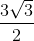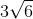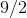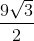Explanation:

The diagonal of a shape is simply the length from a vertex to the center of the face or vertex opposite to it. With a regular tetrahedron, we have a face opposite to the vertex, and this basically amounts to calculating the height of our shape.

We know that the height of a tetrahedron is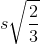where s is the side length, so we can put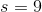into this formula: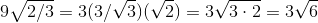which gives us the correct answer.